##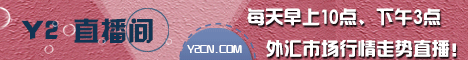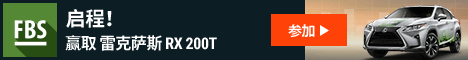# 关于Stochastic Oscillator指标详细解释...求转飞狐公式

0主题 110积分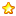2005-12-13发表于 2006-4-22 13:23 | 显示全部楼层 |阅读模式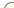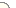Name: Stochastic Oscillator, Stochastic   Author: MetaQuotes Rating: Not rated Downloaded: 223 times Download:   Stochastic.mq4 (3.8 Kb) View Description: The Stochastic Oscillator Technical Indicator compares where a security’s price closed relative to its price range over a given time period. The Stochastic Oscillator is displayed as two lines. The main line is called %K. The second line, called %D, is a Moving Average of %K. The %K line is usually displayed as a solid line and the %D line is usually displayed as a dotted line. There are several ways to interpret a Stochastic Oscillator. Three popular methods include: Buy when the Oscillator (either %K or %D) falls below a specific level (e.g., 20) and then rises above that level. Sell when the Oscillator rises above a specific level (e.g., 80) and then falls below that level; Buy when the %K line rises above the %D line and sell when the %K line falls below the %D line; Look for divergences. For instance: where prices are making a series of new highs and the Stochastic Oscillator is failing to surpass its previous highs. Calculation The Stochastic Oscillator has three variables: %K periods (Pk). This is the number of time periods used in %K calculation. By default is 5; %K Slowing Periods (Sk). This value controls the internal smoothing of %K. A value of 1 is considered a fast stochastic; a value of 3 is considered a slow stochastic. By default is 3; %D periods (Pd). This is the number of time periods used when calculating a moving average of %K. By default is 3; The formula for %K is: %K = 100*SUM (CLOSE - MIN (LOW, Pk), Sk) / SUM (MAX (HIGH, Pk) - MIN (LOW, Pk)), Sk) Where: CLOSE — is today’s closing price; MIN (LOW, Pk) — is the lowest low in Pk periods; MAX (HIGH, Pk) — is the highest high in Pk periods. SUM (CLOSE - MIN (LOW, Pk), Sk) — amount composed CLOSE - MIN (LOW, Pk) for period Sk; SUM (MAX (HIGH, Pk) - MIN (LOW, Pk)), Sk) — amount composed HIGH (Pk)) - MIN (LOW, Pk) for period Sk. The %D moving average is calculated according to the formula: %D = SMA (%K, Pd) Where: Pd — is the smoothing period for %K; SMA — is the Simple Moving Average 以上E文来自于MT4的官方网站，我根据上面的公式导入飞狐，出现调用参数不符的错误，我简单的调整了一下，发现有个括号出现了问题，得出以下的公式 K:SUM(CLOSE-MIN(LOW, Pk),Sk)/SUM(MAX(HIGH, Pk)-MIN(LOW,Pk),Sk); D:SMA(K,Pd,1) 结果显示出的指标与MT4完全不一样！，求高手指点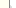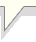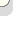0主题 110积分2005-12-13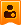楼主| 发表于 2006-4-22 13:34 | 显示全部楼层#property copyright "Copyright ?2004, MetaQuotes Software Corp." #property link      "http://www.metaquotes.net/" #property indicator_separate_window #property indicator_minimum 0 #property indicator_maximum 100 #property indicator_buffers 2 #property indicator_color1 LightSeaGreen #property indicator_color2 Red //---- input parameters extern int KPeriod=5; extern int DPeriod=3; extern int Slowing=3; //---- buffers double MainBuffer[]; double SignalBuffer[]; double HighesBuffer[]; double LowesBuffer[]; //---- int draw_begin1=0; int draw_begin2=0; //+------------------------------------------------------------------+ //| Custom indicator initialization function                         | //+------------------------------------------------------------------+ int init()   {    string short_name; //---- 2 additional buffers are used for counting.    IndicatorBuffers(4);    SetIndexBuffer(2, HighesBuffer);    SetIndexBuffer(3, LowesBuffer); //---- indicator lines    SetIndexStyle(0,DRAW_LINE);    SetIndexBuffer(0, MainBuffer);    SetIndexStyle(1,DRAW_LINE);    SetIndexBuffer(1, SignalBuffer); //---- name for DataWindow and indicator subwindow label    short_name="Sto("+KPeriod+","+DPeriod+","+Slowing+")";    IndicatorShortName(short_name);    SetIndexLabel(0,short_name);    SetIndexLabel(1,"Signal"); //----    draw_begin1=KPeriod+Slowing;    draw_begin2=draw_begin1+DPeriod;    SetIndexDrawBegin(0,draw_begin1);    SetIndexDrawBegin(1,draw_begin2); //----    return(0);   } //+------------------------------------------------------------------+ //| Stochastic oscillator                                            | //+------------------------------------------------------------------+ int start()   {    int    i,k;    int    counted_bars=IndicatorCounted();    double price; //----    if(Bars<=draw_begin2) return(0); //---- initial zero    if(counted_bars<1)      {       for(i=1;i<=draw_begin1;i++) MainBuffer[Bars-i]=0;       for(i=1;i<=draw_begin2;i++) SignalBuffer[Bars-i]=0;      } //---- minimums counting    i=Bars-KPeriod;    if(counted_bars>KPeriod) i=Bars-counted_bars-1;    while(i>=0)      {       double min=1000000;       k=i+KPeriod-1;       while(k>=i)         {          price=Low[k];          if(min>price) min=price;          k--;         }       LowesBuffer=min;       i--;      } //---- maximums counting    i=Bars-KPeriod;    if(counted_bars>KPeriod) i=Bars-counted_bars-1;    while(i>=0)      {       double max=-1000000;       k=i+KPeriod-1;       while(k>=i)         {          price=High[k];          if(maxdraw_begin1) i=Bars-counted_bars-1;    while(i>=0)      {       double sumlow=0.0;       double sumhigh=0.0;       for(k=(i+Slowing-1);k>=i;k--)         {          sumlow+=Close[k]-LowesBuffer[k];          sumhigh+=HighesBuffer[k]-LowesBuffer[k];         }       if(sumhigh==0.0) MainBuffer=100.0;       else MainBuffer=sumlow/sumhigh*100;       i--;      } //---- last counted bar will be recounted    if(counted_bars>0) counted_bars--;    int limit=Bars-counted_bars; //---- signal line is simple movimg average    for(i=0; i
0主题 1228积分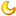2005-12-28发表于 2006-4-22 17:34 | 显示全部楼层飞弧有什么好的0主题 110积分2005-12-13楼主| 发表于 2006-4-22 21:09 | 显示全部楼层至少我要知道这个公式是为什么，怎么来地...0主题 1228积分2005-12-28发表于 2006-4-23 11:51 | 显示全部楼层你如果懂编程,什么怎么来的都知道0主题 110积分2005-12-13楼主| 发表于 2006-4-23 16:10 | 显示全部楼层呵呵！ 不懂所以要多问不是吗？！0主题 710积分2007-9-19发表于 2007-11-20 05:04 | 显示全部楼层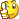本版积分规则 回帖后跳转到最后一页

GMT+8, 2019-5-25 23:03 , Processed in 0.080515 second(s), 28 queries .

Powered by Discuz! X7.2

© 2001-2013 Comsenz Inc.